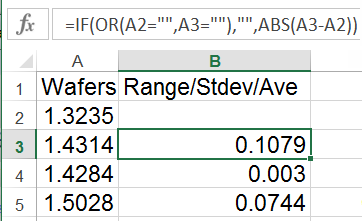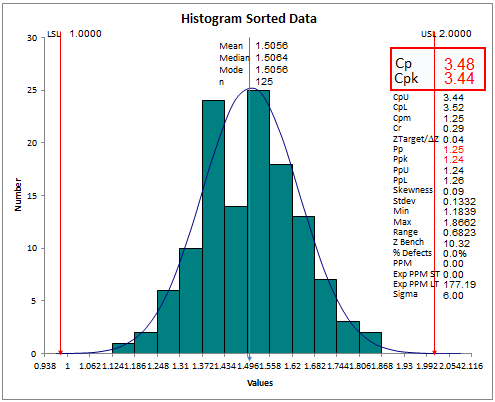# Histogram Common Errors

Over the years, we've gotten a lot of calls about the histogram. Here are a couple of the most common errors users make with their data.

### Sorting Individuals Data

Process capability metrics formulas use the range as part of the sigma estimator formula. Ranges are calculated as the difference between consecutive data points. For example, cell B3 below is the difference between A3 and A2.When you sort the data, it eliminates the natural range between each individual measurement and substitutes an artificial one that's very small. When you do this, Cp and Cpk increase dramatically. Below are two histograms using the same data. The first one has data with normal variation; the second one has sorted data.

Notice the graphs look the same BUT the Cp and Cpk values are different.Tip: When Cp and Pp, or Cpk and Ppk differ dramatically, the data might have been sorted or the process may be too out of control for analysis.

QI Macros mistake proofs your analysis by comparing Cp to Pp and Cpk to Ppk. If the differences are significant, an error box will pop up and the Cp Cpk and Pp Ppk values will be turned red.### Using Individuals Data Instead of Subgroups

Many machines produce single columns of data, but the data is actually in subgroups with a sample size of five. When using subgroups of five, the range or standard deviation is calculated across the five samples. (5 samples uses Sbar/c4 instead of Rbar/d2).

QI Macros contains a prompt when the Histogram detects individuals data. If you see this prompt, enter the number of samples per subgroup (default is 1):Tip: a subgroup is a group of measurements produced under the same set of conditions.

### Using the Wrong Formulas - Cp, Cpk vs Pp, Ppk

Some people still try to calculate Cp and Cpk using standard deviation (stdev), not Sigma estimator. Stdev is used to calculate Pp and Ppk.

### Why Choose QI Macros to Perform Capability Analysis and Draw Histograms?### Affordable

• Only \$329 USD - less with quantity discounts
• No annual fees
• Free Technical Support### Easy to Use

• Works Right in Excel
• Calculates Cp Cpk Pp Ppk
• Accurate Results Without Worry### Proven and Trusted

• 100,000 Users in 80 Countries
• Celebrating 20th Anniversary
• Five Star CNET Rating - Virus Free